Next: Flow Through an Orifice Up: Incompressible Inviscid Flow Previous: Euler Momentum Theorem

Consider a long straight tube through which an inviscid fluid flows at the constant speed. If we place a small obstacle,, in the middle of the tube then the flow in the immediate neighborhood ofwill be modified, but that a great distance upstream or downstream ofwill presumably remain undisturbed. In general, in order to maintain the obstacle at rest, we need to exert both a force and a couple on it. Letbe the component of the force in the direction of the original flow. Neglecting external forces, such as gravity,is the resultant in the direction of the flow of the pressure thrusts acting on the boundary of.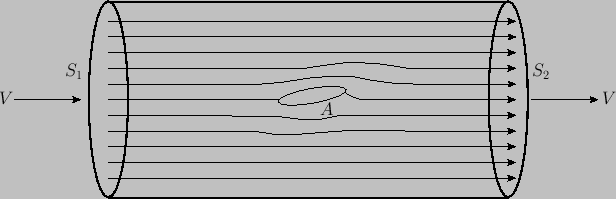Consider two cross-sections,and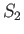, a great distance upstream and downstream of, respectively. The fluid between these sections can be split into a great many stream filaments to each of which Euler's momentum theorem is applicable. The outer filaments are bounded by the walls of the tube, and so the thrust components acting on these are directed perpendicular to the flow. The walls of the filaments in contact withare acted on by the obstacle, which exerts on them a force whose component in the direction of the flow is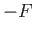. By Euler's theorem, the resultant of all of the thrusts acting on the fluid in the tube is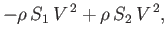(4.11)

which vanishes, because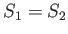.

By Bernoulli's theorem, the pressure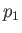acting acrossis the same as the pressureacting across. Thus, according to Euler's theorem,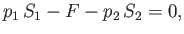(4.12)

which implies that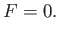(4.13)

The surprising result that the net parallel force exerted by an inviscid fluid stream on a stationary obstacle placed in its path is zero is known as d'Alembert's paradox, after the French scientist Jean-Baptiste d'Alembert (1717-1783).

If we suppose the walls of the tube to recede to infinity then we obtain the case of an obstacle immersed in a moving stream that is unbounded in every direction. The previous proof still shows that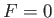.

Finally, if we impose on the whole system a uniform velocityin the direction opposite to that of the stream then the fluid at a great distance is reduced to rest, and the obstaclemoves with the uniform speed. However, superposing a uniform flow does not alter the dynamical conditions. Therefore, we conclude that the resistance to a solid body moving with uniform velocity through an unbounded inviscid fluid, otherwise at rest, is zero.Next: Flow Through an Orifice Up: Incompressible Inviscid Flow Previous: Euler Momentum Theorem
Richard Fitzpatrick 2016-03-31